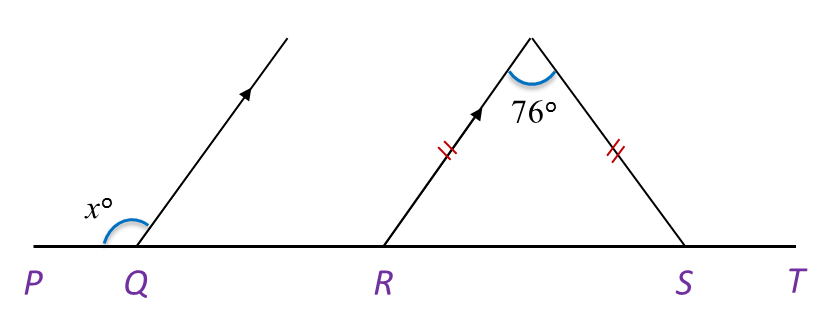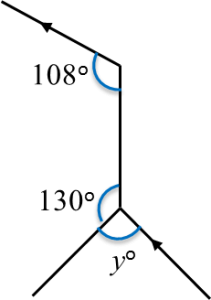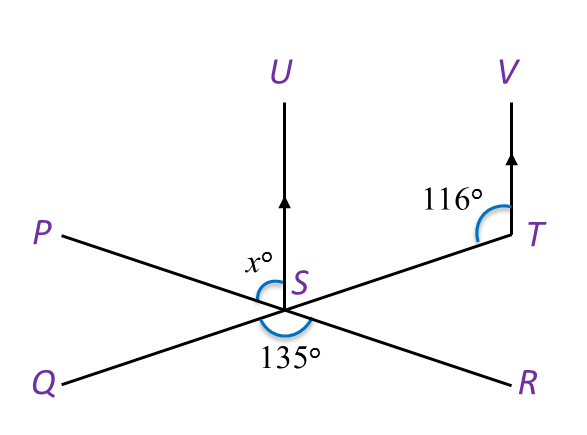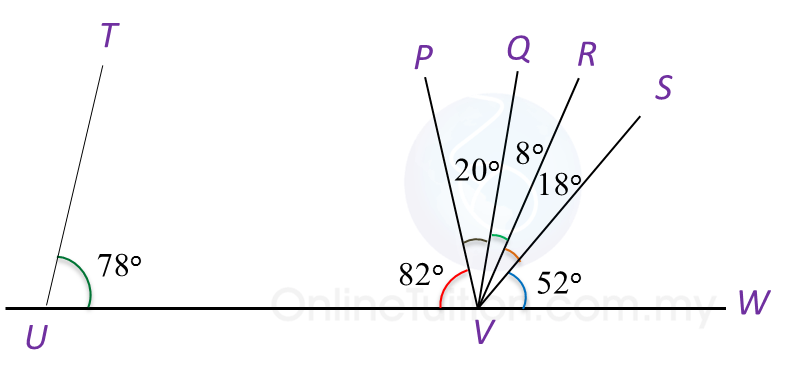# 1.2.2 Angles and Lines II, PT3 Practice

Question 6:
In Diagram below, PQRST is a straight line. Find the value of x.Solution:

Question 7:
In Diagram below, find the value of y.Solution:Question 8:
In Diagram below, PSR and QST are straight lines.Find the value of x.

Solution:
$\begin{array}{l}\angle UST+\angle STV={180}^{o}\\ \angle UST={180}^{o}-{116}^{o}={64}^{o}\\ \angle PST=\angle QSR\\ {x}^{o}+\angle UST={135}^{o}\\ {x}^{o}+\angle UST={135}^{o}\\ {x}^{o}={71}^{o}\\ x=71\end{array}$

Question 9:
In Diagram below, PWV is a straight line.(a) Which line is perpendicular to line PWV?
(b) State the value of  ∠ RWU.

Solution:
(a) SW

(b) ∠ RWU = 13o + 29o + 20o = 62o

Question 10:
In Diagram below, UVW is a straight line.(a) Which line is parallel to line TU?
(b) State the value of  ∠ QVS.

Solution:
(a) QV

(b) ∠ QVS = 8o + 18o = 26o

# 1.2.1 Angles and Lines II, PT3 Practice

 
 
1.2.1 Angles and Lines II, PT3 Practice
 

 
Question 1:
 In Diagram below, PQRS, ABQC and KRL are straight lines.
   
 
Find the value of x.
 
 

  Solution:

   
 
 
 
 
 
 
 
    $\begin{array}{l}\angle KRB+\angle ABR={180}^{o}←\overline{)\text{Sum of interior angles}}\\ \angle KRB+{105}^{o}={180}^{o}\\ \text{}\angle KRB={180}^{o}-{105}^{o}\\ \text{}\angle KRB={75}^{o}\\ \angle BRQ={40}^{o}←\overline{)\text{Corresponding angle}}\\ {x}^{o}=\angle SRL=\angle KRQ←\overline{)\begin{array}{l}\text{Vertically}\\ \text{opposite angle}\end{array}}\\ {x}^{o}={75}^{o}+{40}^{o}\\ \text{}={115}^{o}\\ \text{}x=115\end{array}$
 

 
 
 
 
Question 2:
 (a) In Diagram below, PQR and SQT are straight lines.
   
 
Find the value of x.
 
 

  (b) In Diagram below, PQRS is a straight line. Find the value of x.
   
 
Solution:
 
(a)
    $\begin{array}{l}\angle PQT=\angle RQS\\ {x}^{o}+{90}^{o}={155}^{o}\\ \text{}{x}^{o}={155}^{o}-{90}^{o}\\ \text{}={65}^{o}\\ \text{}x=65\end{array}$  
 
 

  (b)
    $\begin{array}{l}\angle QRT={57}^{o}\\ {x}^{o}+2{x}^{o}={180}^{o}-{57}^{o}\\ \text{3}{x}^{o}={123}^{o}\\ \text{}{x}^{o}=\frac{{123}^{o}}{3}\\ \text{}{x}^{o}={41}^{o}\\ \text{}x=41\end{array}$
 

 
 
 
 
Question 3:
   
 
 
 
    $\begin{array}{l}{x}^{o}=\angle QRT+\angle TRS\\ \angle QRT={40}^{o}\\ \angle TRS={180}^{o}-{135}^{o}\\ \text{}={45}^{o}\\ \text{Hence,}{x}^{o}={40}^{o}+{45}^{o}\\ \text{}{x}^{o}={85}^{o}\\ \text{}x=85\end{array}$  
 
 
 
 
 
Question 4:
   
 
 
 
 
Solution:
 
40o + 80o + xo + xo = 180o
 
2xo = 180o – 120o 
 
2xo = 60o
 
x= 30o
 
x = 30
 
 
 
 
 
 
Question 5:
 
In Diagram below, PQR is an isosceles triangle and QRS is a straight line.
   
 
 Find the values of x and y.
 
 

  Solution:
 
  $\begin{array}{l}\angle PRQ={180}^{o}-{110}^{o}={70}^{o}\\ \angle PRQ=\angle PQR={70}^{o}\\ \text{}{y}^{o}={180}^{o}-{70}^{o}-{70}^{o}\\ \text{}={40}^{o}\\ \text{}y=40\\ {x}^{o}={110}^{o}-{40}^{o}\\ \text{}={70}^{o}\\ \text{}x=70\end{array}$  
 

 

 

# 1.1 Angles and Lines II

PT3 Smart TIP

Alternate angles are easily identified by tracing out the pattern “Z” as shown.

Corresponding angles are easily identified by the pattern “F” as shown.Interior angles are easily identified by the pattern “C” as shown.

Example 1:
Construct a line parallel to PQ and passing through W.
= 40o and b = 50o← (Alternate angles)
= a + b
= 40o+ 50o
= 90o

Example 2:
In Diagram below, PSQ and STU are straight lines. Find the value of x.
$\begin{array}{l}\angle WSQ={180}^{o}-{150}^{o}\\ \text{}={30}^{o}←\overline{)\text{Supplementary angle}}\\ \angle XTU=\angle WSQ+\angle x←\overline{)\text{Corresponding angle}}\\ \text{}{75}^{o}={30}^{o}+\angle x\\ \text{}\angle x={75}^{o}-{30}^{o}\\ \text{}\angle x={45}^{o}\\ \text{}x=45\end{array}$